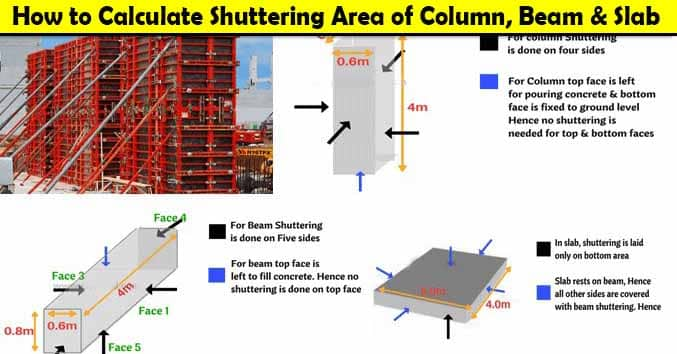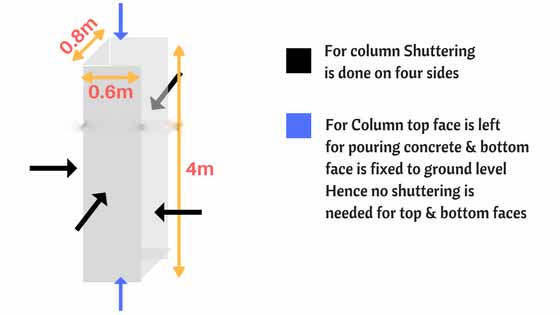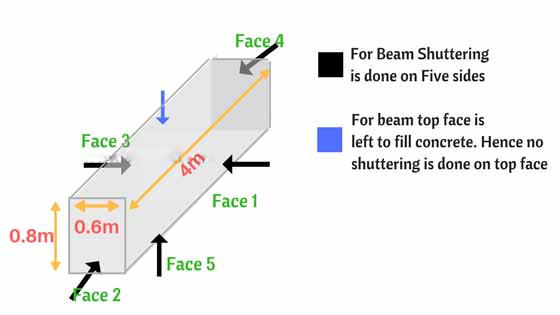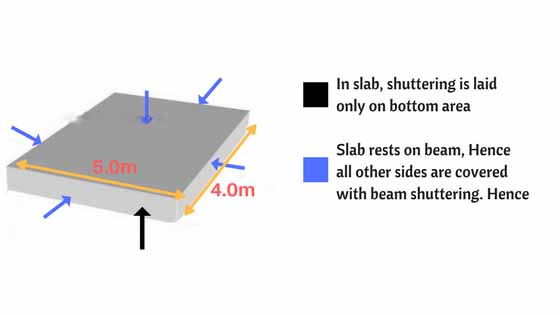# How to Calculate Shuttering Area of Column, Beam and Slab

How to Calculate Shuttering Area, Column Beam and Slab, How to Calculate Shuttering Area of Slab, How to Calculate Shuttering Area of Beam, How to Calculate Shuttering Area of Column, How To Calculate Area of Formwork, Formulae of Shuttering Area

5# How to Calculate Shuttering Area of Column, Beam and Slab | Area of Formwork

In this Article today we will talk about the How to Calculate Shuttering Area | Column Beam and Slab | How to Calculate Shuttering Area of Slab | How to Calculate Shuttering Area of Beam | How to Calculate Shuttering Area of Column | How To Calculate Area of Formwork | Formulae of Shuttering Area

## How to Calculate Shuttering Area of Column, Beam and Slab:

### Shuttering:

Shuttering is an arrangement done for vertical surfaces to support the wet concrete till it attains the desired strength. Shuttering is a part of formwork. Follow below to know how to calculate shuttering area.

### Calculating Shuttering Area:

The shuttering is calculated in terms of Sq.M in the Rate Analysis of Shuttering. In order to calculate area of shuttering you must know how to calculate peripheral length (Perimeter) of an any shape.

Peripheral length (Perimeter):

Perimeter is the distance around a two dimensional shape.

For example square has four sides determine one side length is “s”

then peripheral length  = s+s+s+s = 4s

Important Formulae for Calculating Shuttering Area:

• Perimeter of Square : 4S  (S = Length of Side)
• Perimeter of Rectangle : 2[L+B]  (L=Length & B = Breadth)
• Perimeter of Circle : 2πr  (r = Radius of circle)
• Area of Rectangle = Length x Breadth
• Area of Square = Side x Side

Remember, each member in a structure either it may be Slab or Beam or Column it has six sides (faces). Shuttering area can be calculated by using two methods. One is by below mentioned formula and other method is by calculating the individual areas of faces. To keep it clear, i used both the methods in this article.

### Formulae of Shuttering Area:

Shuttering area = Peripheral length (Perimeter) x Depth

## Calculation of Shuttering Area of a Column:

Consider a column as shown in below figure. To calculate the shuttering area follow the below steps:-For Column, shuttering is done for four sides and other two sides (the top of column is left for filling concrete and bottom is fixed to ground level). Neglect top and bottom in calculation.

The side of column is in rectangle shape with side length “l” and breadth “b”

Peripheral length of Rectangle is   = l+b+l+b = 2l+2b

Shuttering area = Peripheral length (Perimeter) x Depth

Peripheral length = 2×0.8+2×0.6 = 1.6+1.2 = 2.8Sq.m

Total Area of Shuttering of a column  = 2.8 x 4 = 11.2 Sq.m

In case, the shape of column is Circular then the below mentioned fomulae is used for calculating shuttering area

Shuttering area of Circular Column = 2πr  x Depth

## Calculation of Shuttering Area of a Beam:

For calculation purpose i am considering the beam as shown in figure:For Beam, shuttering is done in 5 sides and the other side (top side is left to fill concrete) Shuttering area can also be calculated by finding out the individual area of each faces as below:

• Face 1 : Area of rectangle = L x B = 0.8 x 4 = 3.2
• Face 2 : Area of rectangle = L x B = 0.6 x 4 = 2.4
• Face 3 : Area of rectangle = L x B = 0.8 x 4 = 3.2
• Face 4 : Area of rectangle = L x B = 0.8 x 0.6 = 0.48
• Face 5 : Area of rectangle = L x B = 0.8 x 0.6 = 0.48

Total Area of Shuttering = 3.2 + 2.4 + 3.2+ 0.48 +0.48 = 9.76.Sqm

## Calculation of Shuttering Area of a Slab:

Slab rests on beam, there is no need of providing shuttering to the slab on four sides.  Same as beam & column, the top of the slab is left to fill concrete and for curing. Hence, shuttering is only provided to the bottom of slab. The below mentioned values are considered for finding the shuttering of a slab.Shuttering area of Slab =  Bottom area of slab = L x B Bottom Area =  5 x 4 = 20 Sq.m

## Conclusion:

Full article on Column Beam and Slab | How to Calculate Shuttering Area of Slab | How to Calculate Shuttering Area of Beam | How to Calculate Shuttering Area of Column | How To Calculate Area of Formwork | Formulae of Shuttering Area. Thank you for the full reading of this article in “The Civil Engineering” platform in English. If you find this post helpful, then help others by sharing it on social media. If any formula of BBS is missing from this article please tell me in comments.

1.Farooq Bashir

Thank You, I noted that You are providing very useful technical Informatises to the Viewers.
Money Thanks.

• Thanks for the comments please visit more articles by clicking on “All Posts” tab of the Menu Bar

2.Government service

Thanks

3.Begashaw Takele

good